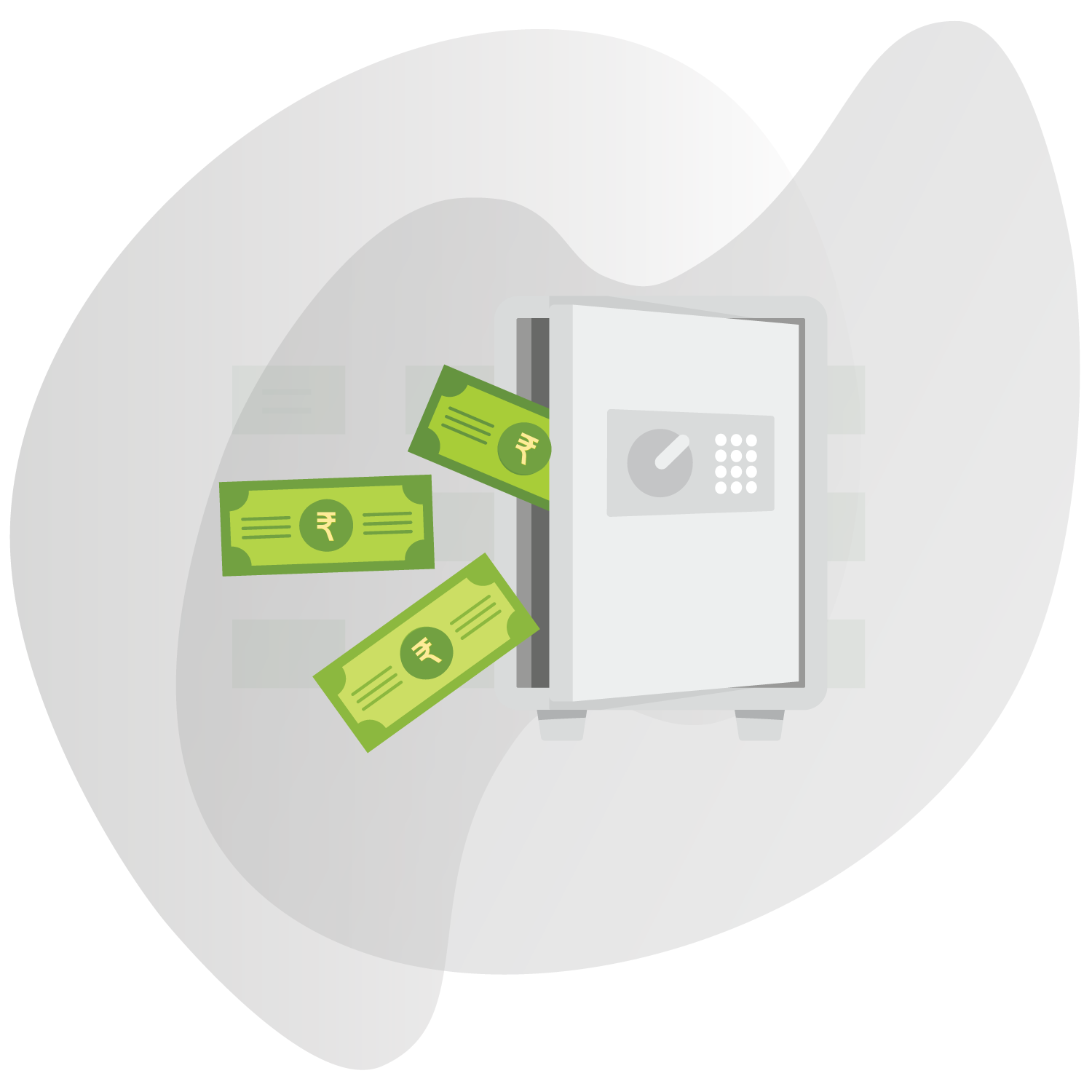# RECURRING DEPOSIT CALCULATOR

Wish to calculate the returns on your RD? Calculate the amount that you would receive at the end by depositing an amount regularly using our Recurring Deposit Calculator.

Monthly Amount Deposited *
Interest Rate(P.A) *
Deposit Tenure:*
YEAR
MONTH#### About Finology Recurring Deposit Calculator

1) What is Recurring Deposit?

Recurring Deposits or RD is an investment instrument offered by various financial institutions, where we can make regular payments and earn interest for the long term. It is like the flexible form of Fixed Deposit because in FD we have to invest in lumpsum but here in Recurring deposit we have to save monthly to earn returns.

2) What are the benefits of Recurring Deposit?

There are various benefits of RD. Some are:

• Better RD Interest rate than normal savings account.
• Acts as a safe investment option.
• Provide generally 7-8% of fixed returns for the period.
• Good option for conservative investors.
• It inculcates the habit of saving.

3) What is the tenure for Recurring Deposit?

The tenure for RD generally ranges from 6 months to 10 years. We can choose it accordingly which satisfies our return needs. Various financial institution provides RD of different maturities and interest rate. The Longer the maturity, higher will be the RD interest rates.

4) How is the RD interest rate calculated?

The RD interest rate is calculated based on its tenure. It is compounded monthly, quarterly, yearly based on the type of option we choose. One does not need to dive into formula of how to calculate RD interest rate. We can directly use the RD interest rate calculator made by finology and enter the sum we want to invest monthly and the tenure, then the interest rate calculator will provide the result of maturity amount and the interest earned. Generally, the RD interest rate is compounded quarterly.

5) Are interest on Recurring Deposits taxable?

Yes, the RD interest rate is taxable as per the Income Tax Act 1961. The tax applicable on RD is deducted at the source by the banks if the total interest exceeds Rs 10000 for the financial year. However, if your total income doesn’t exceed the minimum tax slab then you can avoid the TDS charges by banks on FD by submitting a declaration under section 197A of the Income Tax Act in Form 15-G or 15-H, whichever is applicable.

6) Is Recurring Deposit better than Fixed Deposit?

Both FD and RD are a safe investment option with a fixed maturity period, the only difference is that in FD we have to invest in lumpsum but in RD we can deposit monthly. Due to this the return also varies in both. An investor can choose any among them depending upon his needs, i.e., if he doesn’t have lumpsum income then Recurring Deposit is the best option.

7) How to use this online Recurring Deposit calculator?

We have made a RD calculator online which can help you know your maturity and interest amount quickly without any formulas. You just need to enter the amount deposited in the RD monthly, the Interest rate which is offered and the tenure. After providing these values our RD interest rate calculator will show the amount which you will get at maturity and the total interest which is earned on it through quarterly compounding.